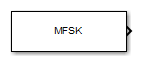# MFSK Waveform

Multiple frequency shift keying (MFSK) continuous waveform

## Library

Waveforms

`phasedwavlib`

•## Description

The MFSK Waveform block generates a multiple frequency- shift keying (MFSK) continuous waveform with a specified step time, sweep bandwidth, frequency offset, and number of steps. The block outputs an integer number of samples, steps, or sweeps. For details on the structure of an MFSK waveform, see `phased.MFSKWaveform`.

## Parameters

Sample rate (Hz)

Sample rate of the signal, specified as a positive scalar. Units are in hertz.

Sweep bandwidth (Hz)

Bandwidth of the MFSK sweep, specified as a positive scalar. Units are in hertz.

Frequency step burst time (s)

Time duration of each frequency step, specified as a positive scalar. Units are in seconds.

Number of steps per sweep

Total number of steps in each sweep, specified as an even positive integer.

Chirp offset frequency (Hz)

Chirp offset frequency, specified as a real scalar. Units are in hertz. The offset determines the frequency translation between the two sequences.

Output signal format

Format of the output signal, specified as one of the following:

• `'Steps'` — The block outputs the number of samples contained in an integer number of frequency steps, Number of steps in output.

• `'Samples'` — The block outputs the number of samples specified in Number of samples in output.

• `'Sweeps'` — The block outputs the number of samples contained in an integer number of sweeps, Number of sweeps in output.

Number of sweeps in output

Number of sweeps in the block output, specified as a positive integer. This parameter appears only when you set Output signal format to `Sweeps`.

Number of samples in output

Number of samples in the block output, specified as a positive integer. This parameter appears only when you set Output signal format to `Samples`.

Number of steps in output

Number of steps in the block output, specified as a positive integer. This parameter appears only when you set Output signal format to `Steps`.

Simulate using

Block simulation method, specified as `Interpreted Execution` or ```Code Generation```. If you want your block to use the MATLAB® interpreter, choose `Interpreted Execution`. If you want your block to run as compiled code, choose `Code Generation`. Compiled code requires time to compile but usually runs faster.

Interpreted execution is useful when you are developing and tuning a model. The block runs the underlying System object™ in MATLAB. You can change and execute your model quickly. When you are satisfied with your results, you can then run the block using ```Code Generation```. Long simulations run faster than they would in interpreted execution. You can run repeated executions without recompiling. However, if you change any block parameters, then the block automatically recompiles before execution.

When setting this parameter, you must take into account the overall model simulation mode. The table shows how the Simulate using parameter interacts with the overall simulation mode.

When the Simulink® model is in `Accelerator` mode, the block mode specified using Simulate using overrides the simulation mode.

Acceleration Modes

 Block Simulation Simulation Behavior `Normal` `Accelerator` `Rapid Accelerator` `Interpreted Execution` The block executes using the MATLAB interpreter. The block executes using the MATLAB interpreter. Creates a standalone executable from the model. `Code Generation` The block is compiled. All blocks in the model are compiled.

## Ports

### Note

The block input and output ports correspond to the input and output parameters described in the `step` method of the underlying System object. See link at the bottom of this page.

PortSupported Data Types
`Out`Double-precision floating point